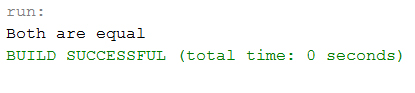## Java Programming Tutorial Index

else if statements in Java is like another if condition, it's used in the program when if statement having multiple decisions.

The basic format of else if statement is:

Syntax:

``````if(test_expression)
{
}
else if(test_expression n)
{
}
else
{
}``````

### Example of a Java Program to Demonstrate else If statements

Example:

``````public class Sample {

public static void main(String args[]) {
int a = 30, b = 30;

if (b > a) {
System.out.println("b is greater");
}
else if(a > b){
System.out.println("a is greater");
}
else {
System.out.println("Both are equal");
}
}
}``````

Program Output: